MECH ENGG » APM WINMACHINE » APM DYNAMICS

# APM Dynamics

APM Dynamics - a product designed to solve the problems of modeling the dynamics of spatial mechanical systems by the finite element method (FEM).

The application of the MCE made it possible to abandon the representation of dynamical systems in the form of absolutely rigid bodies, as assumed in classical mechanics, and take into account the elastic properties of these bodies. The elastic properties of dynamical systems are automatically formed, which greatly simplifies the practice of applying the proposed method.

The geometry of the elements of mechanical systems is created with the help of a modern three-dimensional graphic editor, in which there are both tools for geometric modeling, and means for specifying boundary conditions and sensors for recording the required output parameters.

The dynamic model is formed in the form of one-, two-, three- and n-mass systems (n is an arbitrary integer) and systems with distributed masses.

New algorithms have been developed to simulate the dynamics of inelastic mechanical systems. Equations of motion are effectively solved by the method of decomposition into its own forms, which has the following possibilities:

• provides acceptable accuracy of calculations
• allows us to solve problems of large dimension
• reduces calculation time to achieve the appropriate accuracy in comparison with known solutions, such as the direct integration method (Wilson method), etc.

The proposed numerical approaches allowed us to describe satisfactorily the transient processes that occur during the movement of mechanisms and machines, which can not be achieved with the traditional representation of dynamic objects in the form of absolutely rigid bodies.

Realized solutions allow correctly describe the laws of motion in three-dimensional space as spatial mechanisms, as well as arbitrary mechanical systems.

APM Dynamics includes the following tools:

• A preprocessor for describing the geometry of the model, including procedures for specifying the boundary conditions imposed on the node points
• A preprocessor for specifying the laws of motion of the leading links and force factors acting on the elements of the mechanical system (components of spatial forces and moments)
• Means for specifying flywheels for a more accurate description of the inertial properties of spatial mechanisms
• Solver, implementing the proposed methods for solving dynamic problems
• Postprocessor for visualization and printing of calculation results for linear and angular displacements, linear and angular velocities and accelerations, trajectories of arbitrary points of the design model, current force factors acting on the elements of the mechanical system
• Mechanisms of the animation representation of the motion of system elements in three-dimensional space
• Tools and formats for transferring data, including dynamic loads, to the strength analysis module APM Structure3D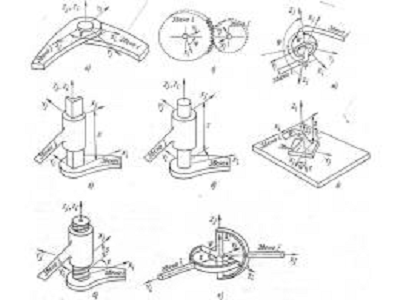JOINT PAIR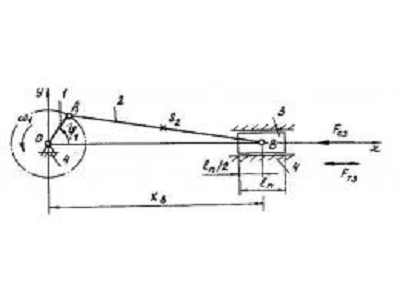CRANK SLIDER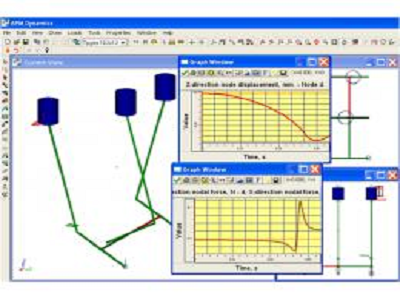MULTIPLE CRANK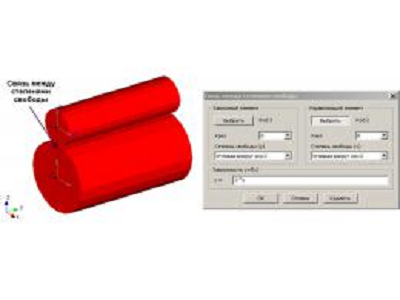GEAR DRIVE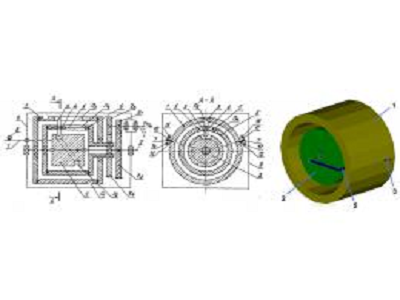INTERNAL DRIVE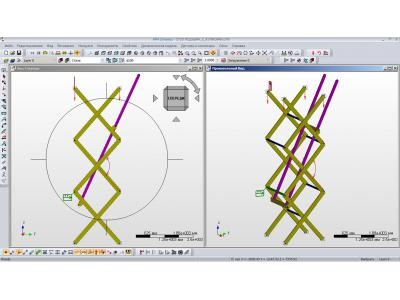SCISSOR LIFT
info@paviathintegratedsolution.com www.paviathintegratedsolution.com